# Ex.6.3 Q6 Squares and Square Roots Solutions - NCERT Maths Class 8

Go back to  'Ex.6.3'

## Question

For each of the following numbers, find the smallest whole number by which it should be divided so as to get a perfect square. Also find the square root of the square number so obtained.

(i) $$252$$

(ii) $$2925$$

(iii) $$396$$

(iv) $$2645$$

(v) $$2800$$

(vi)$$1620$$

## Text Solution

What is known?

Numbers

What is unknown?

The smallest whole number by which it should be divided so as to get a perfect square number.

Reasoning:

To get a perfect square, each factor of the given number must be paired.

Steps:

(i) $$252$$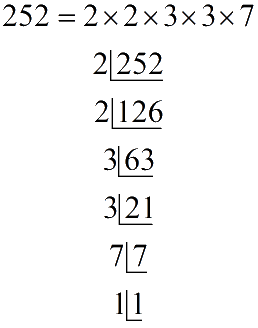Hence, prime factor $$7$$ does not have its pair. If number is divided by $$7$$ then rest of the prime factor will be in pairs.

Therefore, $$252$$ has to be divided by $$7$$ to get a perfect square.

$252 \div 7 = 36$

$$36$$ is perfect square

\begin{align}36 &= 2 \times 2 \times 3 \times 3\\ &= {2^2} \times {3^2}\\ &= {(2 \times 3)^2}\\\sqrt {36} &= 2 \times 3 = 6\end{align}

(ii) $$2925$$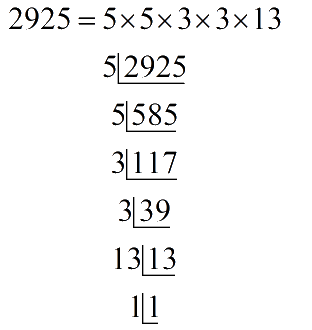Hence, prime factor $$13$$ does not have its pair. If number is divided by $$13$$ then rest of the prime factor will be in pairs.

Therefore, $$2925$$ has to be divided by $$13$$ to get a perfect square.

$2925 \div 13 = 225$

$$225$$ is a perfect square

\begin{align}225 &= 5 \times 5 \times 3 \times 3\\&= {5^2} \times {3^2}\\&= {(5 \times 3)^2}\\\sqrt {225}&= 15\end{align}

(iii) $$396$$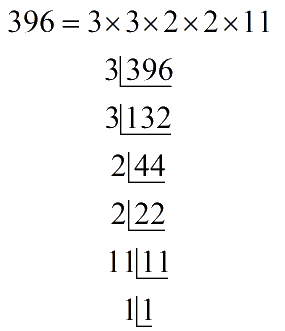Hence, prime factor $$11$$ does not have its pair. If number is divided by $$11$$ then rest of the prime factor will be in pairs. Therefore, $$396$$ has to be divided by $$11$$ to get a perfect square.

\begin{align} 396 \div 11 &= 36\\ 36 &= 3 \times 3 \times 2 \times 2\\&= {3^2} \times {2^2}\\&= {(3 \times 2)^2}\\\sqrt {36}&= 3 \times 2 = 6\end{align}

$$36$$ isa perfect square

(iv) $$2645$$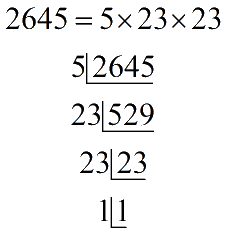Hence, prime factor $$5$$ does not have its pair. If number is divided by $$5$$ then rest of the prime factor will be in pairs. Therefore, $$2645$$ has to be divided by $$5$$ to get a perfect square.

$2645 \div 5 = 529$

$$592$$ is a perfect square

\begin{align}529 &= 23 \times 23\\&= {23^2}\\\sqrt {529}&= 23\end{align}

(v) $$2800$$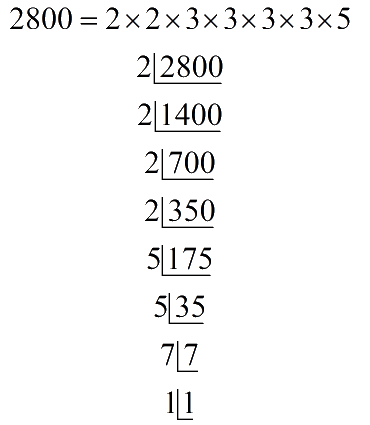Hence, prime factor $$7$$ does not have its pair. If number is divided by $$7$$ then rest of the prime factor will be in pairs. Therefore, $$2800$$ has to be divided by $$7$$ to get a perfect square

$2800 \div 7 = 400$

$$400$$ is a perfect square

\begin{align}400 &= 2 \times 2 \times 2 \times 2 \times 5 \times 5\\ &= {2^2} \times {2^2} \times {5^2}\\ &= {(2 \times 3 \times 5)^2}\\\sqrt {400} &= 2 \times 2 \times 5\\ &= 20\end{align}

(vi) $$1620$$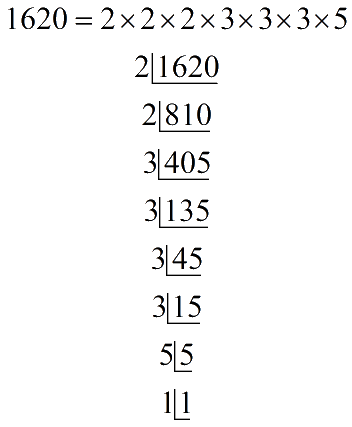Hence, prime factor $$5$$ does not have its pair. If number is divided by $$5$$ then rest of the prime factor will be in pairs. Therefore, $$1620$$ has to be divided by $$5$$ to get a perfect square.

$1620 \div 5 = 324$

$$324$$ is a perfect square

\begin{align}324 &= 2 \times 2 \times 3 \times 3 \times 3 \times 3\\&= {2^2} \times {3^2} \times {3^2}\\ &= {(2 \times 3 \times 3)^2}\\\sqrt {324}&= 2 \times 3 \times 3\\&= 18\end{align}

Learn from the best math teachers and top your exams

• Live one on one classroom and doubt clearing
• Practice worksheets in and after class for conceptual clarity
• Personalized curriculum to keep up with school